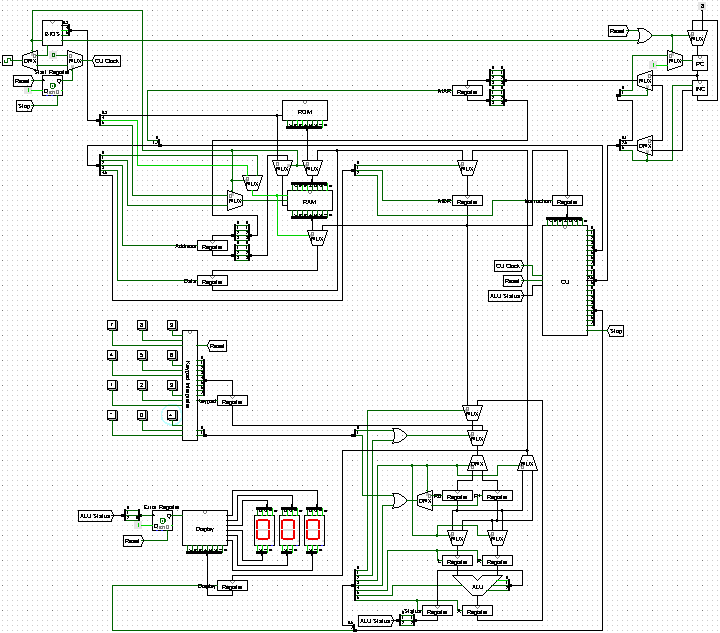# Set up a localhost Web Server for Faster Website Development

January 29, 2022

Two approaches: DNS approach and hosts file approach. The DNS approach is more convenient as it doesn’t require modifications to the hosts file and virtual host config file each time a new virtual host is added. The small catch is that the DNS approach relies on third party service such as `http://localhost.tv/`.

## DNS Approach

### 1. Enable virtual host alias and virtual host config

In Apache `conf` folder, open `httpd.conf`, uncomment `LoadModule vhost_alias_module modules/mod_vhost_alias.so`. Also uncomment `Include conf/extra/httpd-vhosts.conf`.

### 2. Config virtual host

In Apache `conf\extra` folder, open `httpd-vhosts.conf`, add the following lines:

``````<VirtualHost *:80>
ServerName catchall.localhost.tv
ServerAlias *.localhost.tv
VirtualDocumentRoot "C:\Users\[user]\Documents\%1"
<Directory "C:\Users\[user]\Documents">
Require all granted
</Directory>
</VirtualHost>
``````

Only need to do this once. `%1` means the first part of dot-separated components of the server name, i.e. `*`.

### 3. Access the virtual host

Create any folder, e.g. `[site]` under the virtual root `C:\Users\[user]\Documents`. Place an `index.html` file in the folder. It can then be access via `[site].localhost.tv`.

## hosts File Approach

### 1. Config hosts file

Open Notepad as administrator, open `C:\Windows\System32\drivers\etc\hosts`.

In the hosts file, add the following line: `127.0.0.1 site1.localhost`

Repeat for other virtual servers.

### 2. Enable virtual host config

In Apache `conf` folder, open `httpd.conf`, uncomment `Include conf/extra/httpd-vhosts.conf`.

### 3. Config virtual host

In Apache `conf\extra` folder, open `httpd-vhosts.conf`, add the following lines:

``````<VirtualHost *:80>
ServerName site1.localhost
DocumentRoot "C:\Users\[user]\Documents\site1"
<Directory "C:\Users\[user]\Documents\site1">
Require all granted
</Directory>
</VirtualHost>
``````

### 4. Access the virtual host

Create a folder `site1` under the virtual root `C:\Users\[user]\Documents`. Place an `index.html` file in the folder. It can then be access via `site1.localhost`.

To add another virtual host say `site2.localhost`, repeat step 1 and step 2 and add entries for `site2` accordingly.

June 16, 2021

June 20, 2019

# aaencode - Obfuscate JavaScript by Emoticons

November 22, 2017
``````ﾟωﾟﾉ= /｀ｍ´）ﾉ ~┻━┻   //*´∇｀*/ ['_']; o=(ﾟｰﾟ)  =_=3; c=(ﾟΘﾟ) =(ﾟｰﾟ)-(ﾟｰﾟ); (ﾟДﾟ) =(ﾟΘﾟ)= (o^_^o)/ (o^_^o);(ﾟДﾟ)={ﾟΘﾟ: '_' ,ﾟωﾟﾉ : ((ﾟωﾟﾉ==3) +'_') [ﾟΘﾟ] ,ﾟｰﾟﾉ :(ﾟωﾟﾉ+ '_')[o^_^o -(ﾟΘﾟ)] ,ﾟДﾟﾉ:((ﾟｰﾟ==3) +'_')[ﾟｰﾟ] }; (ﾟДﾟ) [ﾟΘﾟ] =((ﾟωﾟﾉ==3) +'_') [c^_^o];(ﾟДﾟ) ['c'] = ((ﾟДﾟ)+'_') [ (ﾟｰﾟ)+(ﾟｰﾟ)-(ﾟΘﾟ) ];(ﾟДﾟ) ['o'] = ((ﾟДﾟ)+'_') [ﾟΘﾟ];(ﾟoﾟ)=(ﾟДﾟ) ['c']+(ﾟДﾟ) ['o']+(ﾟωﾟﾉ +'_')[ﾟΘﾟ]+ ((ﾟωﾟﾉ==3) +'_') [ﾟｰﾟ] + ((ﾟДﾟ) +'_') [(ﾟｰﾟ)+(ﾟｰﾟ)]+ ((ﾟｰﾟ==3) +'_') [ﾟΘﾟ]+((ﾟｰﾟ==3) +'_') [(ﾟｰﾟ) - (ﾟΘﾟ)]+(ﾟДﾟ) ['c']+((ﾟДﾟ)+'_') [(ﾟｰﾟ)+(ﾟｰﾟ)]+ (ﾟДﾟ) ['o']+((ﾟｰﾟ==3) +'_') [ﾟΘﾟ];(ﾟДﾟ) ['_'] =(o^_^o) [ﾟoﾟ] [ﾟoﾟ];(ﾟεﾟ)=((ﾟｰﾟ==3) +'_') [ﾟΘﾟ]+ (ﾟДﾟ) .ﾟДﾟﾉ+((ﾟДﾟ)+'_') [(ﾟｰﾟ) + (ﾟｰﾟ)]+((ﾟｰﾟ==3) +'_') [o^_^o -ﾟΘﾟ]+((ﾟｰﾟ==3) +'_') [ﾟΘﾟ]+ (ﾟωﾟﾉ +'_') [ﾟΘﾟ]; (ﾟｰﾟ)+=(ﾟΘﾟ); (ﾟДﾟ)[ﾟεﾟ]='\\'; (ﾟДﾟ).ﾟΘﾟﾉ=(ﾟДﾟ+ ﾟｰﾟ)[o^_^o -(ﾟΘﾟ)];(oﾟｰﾟo)=(ﾟωﾟﾉ +'_')[c^_^o];(ﾟДﾟ) [ﾟoﾟ]='\"';(ﾟДﾟ) ['_'] ( (ﾟДﾟ) ['_'] (ﾟεﾟ+(ﾟДﾟ)[ﾟoﾟ]+ (ﾟДﾟ)[ﾟεﾟ]+(ﾟΘﾟ)+ (ﾟｰﾟ)+ (ﾟΘﾟ)+ (ﾟДﾟ)[ﾟεﾟ]+(ﾟΘﾟ)+ ((ﾟｰﾟ) + (ﾟΘﾟ))+ (ﾟｰﾟ)+ (ﾟДﾟ)[ﾟεﾟ]+(ﾟΘﾟ)+ (ﾟｰﾟ)+ ((ﾟｰﾟ) + (ﾟΘﾟ))+ (ﾟДﾟ)[ﾟεﾟ]+(ﾟΘﾟ)+ ((o^_^o) +(o^_^o))+ ((o^_^o) - (ﾟΘﾟ))+ (ﾟДﾟ)[ﾟεﾟ]+(ﾟΘﾟ)+ ((o^_^o) +(o^_^o))+ (ﾟｰﾟ)+ (ﾟДﾟ)[ﾟεﾟ]+((ﾟｰﾟ) + (ﾟΘﾟ))+ (c^_^o)+ (ﾟДﾟ)[ﾟεﾟ]+(ﾟｰﾟ)+ ((o^_^o) - (ﾟΘﾟ))+ (ﾟДﾟ)[ﾟεﾟ]+(ﾟΘﾟ)+ (ﾟΘﾟ)+ (c^_^o)+ (ﾟДﾟ)[ﾟεﾟ]+(ﾟΘﾟ)+ (ﾟｰﾟ)+ ((ﾟｰﾟ) + (ﾟΘﾟ))+ (ﾟДﾟ)[ﾟεﾟ]+(ﾟΘﾟ)+ ((ﾟｰﾟ) + (ﾟΘﾟ))+ (ﾟｰﾟ)+ (ﾟДﾟ)[ﾟεﾟ]+(ﾟΘﾟ)+ ((ﾟｰﾟ) + (ﾟΘﾟ))+ (ﾟｰﾟ)+ (ﾟДﾟ)[ﾟεﾟ]+(ﾟΘﾟ)+ ((ﾟｰﾟ) + (ﾟΘﾟ))+ ((ﾟｰﾟ) + (o^_^o))+ (ﾟДﾟ)[ﾟεﾟ]+((ﾟｰﾟ) + (ﾟΘﾟ))+ (ﾟｰﾟ)+ (ﾟДﾟ)[ﾟεﾟ]+(ﾟｰﾟ)+ (c^_^o)+ (ﾟДﾟ)[ﾟεﾟ]+(ﾟΘﾟ)+ (ﾟΘﾟ)+ ((o^_^o) - (ﾟΘﾟ))+ (ﾟДﾟ)[ﾟεﾟ]+(ﾟΘﾟ)+ (ﾟｰﾟ)+ (ﾟΘﾟ)+ (ﾟДﾟ)[ﾟεﾟ]+(ﾟΘﾟ)+ ((o^_^o) +(o^_^o))+ ((o^_^o) +(o^_^o))+ (ﾟДﾟ)[ﾟεﾟ]+(ﾟΘﾟ)+ (ﾟｰﾟ)+ (ﾟΘﾟ)+ (ﾟДﾟ)[ﾟεﾟ]+(ﾟΘﾟ)+ ((o^_^o) - (ﾟΘﾟ))+ (o^_^o)+ (ﾟДﾟ)[ﾟεﾟ]+(ﾟΘﾟ)+ (ﾟｰﾟ)+ (o^_^o)+ (ﾟДﾟ)[ﾟεﾟ]+(ﾟΘﾟ)+ ((o^_^o) +(o^_^o))+ ((o^_^o) - (ﾟΘﾟ))+ (ﾟДﾟ)[ﾟεﾟ]+(ﾟΘﾟ)+ ((ﾟｰﾟ) + (ﾟΘﾟ))+ (ﾟΘﾟ)+ (ﾟДﾟ)[ﾟεﾟ]+(ﾟΘﾟ)+ ((o^_^o) +(o^_^o))+ (c^_^o)+ (ﾟДﾟ)[ﾟεﾟ]+(ﾟΘﾟ)+ ((o^_^o) +(o^_^o))+ (ﾟｰﾟ)+ (ﾟДﾟ)[ﾟεﾟ]+(ﾟｰﾟ)+ ((o^_^o) - (ﾟΘﾟ))+ (ﾟДﾟ)[ﾟεﾟ]+((ﾟｰﾟ) + (ﾟΘﾟ))+ (ﾟΘﾟ)+ (ﾟДﾟ)[ﾟoﾟ]) (ﾟΘﾟ)) ('_');
``````

# How to Force HTTPS Using the .htaccess

November 13, 2017

Long story short, I purchased a domain and hosted it with FastComet a week ago. After setting up the SSL/TLS, I tried to force all web traffic to use HTTPS. One way of achieving this would be adding the following code in the .htaccess file in the website’s root folder.

# Three Modes of Thinking

September 10, 2017
• Logical thinking - Mathematics (inference)
• Empirical thinking - Physics (observation, induction)
• Computational thinking - Computer Science (abstraction, automation)

# COMP 273 CPU Project Redo

August 31, 2017# Watch Out for the Auto Line Ending Conversions

August 26, 2017

Yesterday, I noticed that in some of the files in my local repository, the line breaks were changed from UNIX style LF to Windows style CRLF. The problem occurred after I made some changes on the remote repository GitHub, and later on pulled them to my local repository using GitHub for Windows.

# Two Anti-Spam Measures Against Email Address Harvesting

August 25, 2017

Let’s say we want to obfuscate the following mailto link:

# Up and Running with Jekyll

August 24, 2017

These tools have been used to set up my blog: